## 2. 5. 3 Accumulation

In many cases it is important to obtain the result of a sum of all values of a certain function (or quantity), which are incident to an element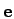. For the sake of explicitness, the topological elementdenotes the traversed element and fulfills the condition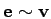, wheredenotes the incidence relation. It is clear that a method for finding all elements of fulfilling the condition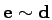has to be given by the topological framework. Usually the underlying framework provides traversal for incidence and adjacency relations as well as the traversal of all elements in a cell complex.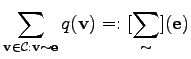(2.39)

It has to be stated that the functional character of the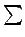symbol is different. On the left hand side of (2.39) thesymbol denotes a summation in the commonly used sense, which is passed an expression (here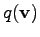) as well as a summation range, namely all elements for whichholds true. On the right hand side, thesymbol denotes a function which is passed the base element of the traversal as well as a function which is applied to all elements to be traversed. The sum of the respective function results is returned as function value of the summation function.

In this notation there is no explicit use of the traversed element, whose explicit naming is not necessary, because it is clear that all evaluations of the function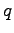are performed for which the relation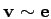holds true. In many cases the summation is not used on the very general incidence relation but on a restricted version which can be written in terms of the traversal function.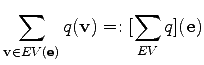(2.40)

The main advantage of this functional formulation is that the formulation of the expression is free from the given argument. This eases the effort of specification when coding the formulae and makes the resulting code less error prone.

Michael 2008-01-16